# How to Exclude a Cell in Excel AVERAGE Formula (4 Methods)

Get FREE Advanced Excel Exercises with Solutions!

In Microsoft Excel, we use the AVERAGE formula to determine the average of specific values from a data range. To extract the exact value of the average, we need to know how to exclude a cell in the excel AVERAGE formula. Because sometimes dataset contains zero or blank cells and excel takes that cell into account to calculate the average value. So, it gives a deviation from the actual value of the average that we intend.

## 4 Methods to Exclude a Cell in Excel AVERAGE Formula

In this article, we will explain 4 methods to exclude a cell in the excel AVERAGE formula. To illustrate these methods to you we may use different datasets for different methods. We will go over several examples to clarify all the methods in this article.

### 1. Input Argument Manually to Exclude a Cell in Excel AVERAGE Formula

The easiest and simplest method to exclude a cell in the excel AVERAGE formula is to input argument manually in the AVERAGE formula. As we input the arguments manually so it’s easy to exclude cells that we don’t want to include in the AVERAGE formula. In the following dataset, we have Sales Amounts for different persons on different days of the week. We can see there are zero and blank cells in the dataset. If we input range (B5:E15) as the argument of the AVERAGE formula it will calculate the average considering the zero and blank cells. But we want to excuse zero and blank cells from the calculation of average.So, let’s take a look at the steps to Input Argument Manually to Exclude a Cell in Excel AVERAGE Formula.

STEPS:

• First, select cell D12 where we want to return the average value.
• Next, insert the following formula in that cell:
`=AVERAGE(C6,E6,C7,D7,E8,D9,C10,E10)`
• To exclude the zero and blank cells hold the Ctrl key and select the cells that you want to take as arguments in the AVERAGE formula.
• After that, press Enter.• Finally, the above actions give us the average value only for selected cells in cell D12. So, with the manual selection of arguments, we can easily exclude cells from the AVERAGE formula.### 2. Ignore Blank/Zero Cells to Exclude a Cell in Excel AVERAGE Formula

In this example, we will see how to deal with zero and blank cells in our excel worksheet. To clarify the motive of this method, we will calculate the average of the following dataset for two cases. In the first case, we will exclude only the blank cells and will input the calculated average value in cell D13. On the other hand, for the second case, we will exclude both zero and blank cells and input the calculated average value in cell D14.Now, let’s take a look at the steps Ignore Blank/Zero Cells in Excel AVERAGE Formula.

STEPS:

• Firstly, select cell D13.
• Next, insert the following formula in that cell:
`=AVERAGE(C6:E10)`• Then, press Enter.So, we get the average value for the value range (C6:E10). If we notice the above calculation, the AVERAGE formula excludes the blank cells by default. But it considers zero cells. That’s why the average value is different from the previous example.

• Secondly, we will move to our second case. Go to the File tab.• Next, select Options.• Then select the option Advanced from the newly appeared dialogue box. Scroll down and uncheck the option “Show a zero in cells that have zero value” from the section “Display options for this worksheet”.
• Now, press OK.• So, we can see this will remove the zero values from the dataset.• Thirdly, select cell E14 and insert the following formula in that cell:
`=AVERAGE(C6:E10)`• Then press Enter.
• Lastly, we can see the result is the same for both cases. So, if we have zero values in the dataset, we have to delete them manually to exclude them from the calculation of the AVERAGE formula.### 3. Use AVERAGE Formula for Ignoring Error Cells

Sometimes we will have error values in our dataset. If we include that error cells in our excel formula we will get an error in our result also. In the following dataset, we have three error cells. To clarify this method to you firstly we will calculate the average value for the data range including error cells. Secondly, we will do the same but this time we will exclude error cells with the IFERROR function.So, let’s see the steps to Use the AVERAGE Formula for Ignoring Error Cells in Excel.

STEPS:

• In the beginning select cell D13 and insert the following formula:
`=AVERAGE(C6:E10)`
• Then press Enter.• So, we can see that the above command returns an error in cell D13.• Next, select cell D14 and insert the following formula:
`=AVERAGE(IFERROR(C6:E10,""))`
• Now, press Enter.• Finally, the error cells are ignored. We get the average value excluding error cells in cell D14.🔎 How Does the Formula Work?

• IFERROR(C6:E10,””): This part checks if there are any error values in the data range (C6:C10) and returns values excluding error cells.
• AVERAGE(IFERROR(C6:E10,””)): Returns the average value for the cells in the data range (C6:C10) excluding error cells.

### 4. Combination of Excel SUM and COUNT Functions to Exclude Cells

In this example, we will use a combination of SUM and COUNT functions to exclude a cell in the AVERAGE formula. In the following dataset, we will calculate the average sales for different days of weeks. At the time of calculating the average, we will exclude the cells containing zero values.

##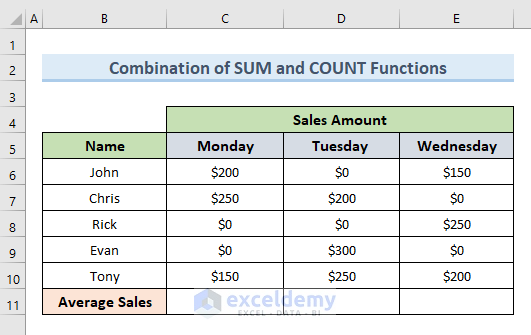Let’s see how we can do this by following some simple steps.

STEPS:

• Firstly, select cell C11 and insert the following formula:
`=(SUM(C6:C10)-SMALL(C6:C10,1)-SMALL(C6:C10,2))/(COUNT(C6:C10)-2)`
• Secondly, press Enter.

##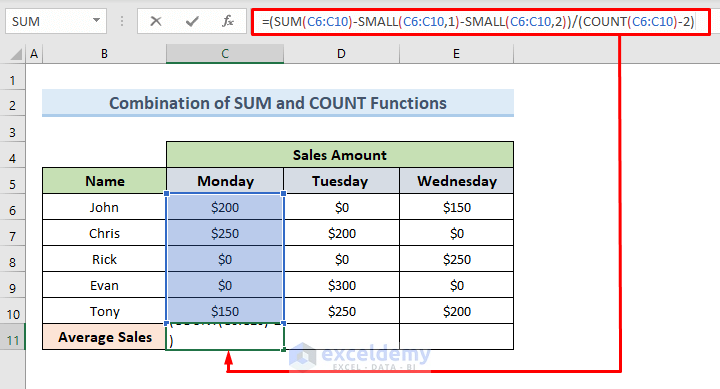• So, the above command returns the average value on Monday in cell C13. If we notice we have excluded the zero cells from our calculation.

##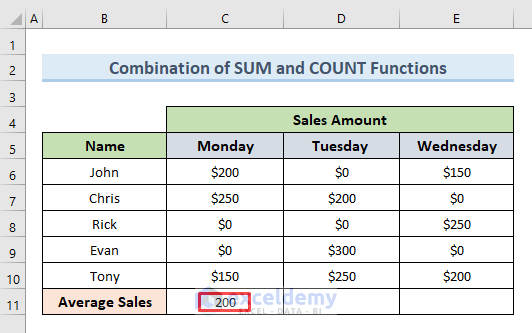• Thirdly, select cell C13 and drag the Fill Handle tool horizontally to cell E11.

##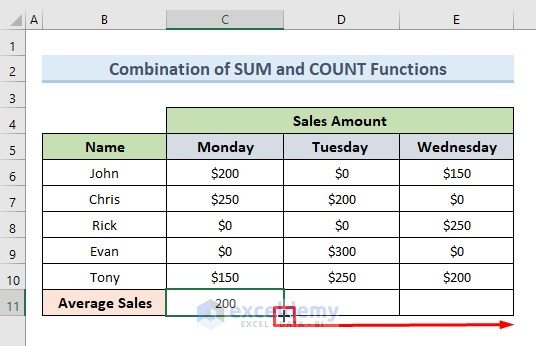• The above action will copy the formula of cell C13 in cells D13 & E13 and returns the average values of Sales Amount for Tuesday and Wednesday.

##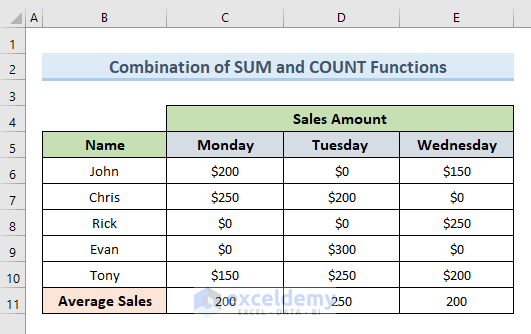🔎 How Does the Formula Work?

• COUNT(C6:C10)-2): Counts total cell numbers from C6 to C10 and ignores the lowest two cells which are zero cells.
• SUM(C6:C10): Returns the totals for cells C6 to C10.
• SUM(C6:C10)-SMALL(C6:C10,1)-SMALL(C6:C10,2): Here the SMALL function determines the lowest two parts of the value range (C6:C10). Then the lowest two values are subtracted from the total.
• SUM(C6:C10)-SMALL(C6:C10,1)-SMALL(C6:C10,2))/(COUNT(C6:C10)-2: Returns the average excluding two zero cells.

Read More: How to Calculate 7 Day Moving Average in Excel (4 Ways)

## Related Articles#### Mukesh Dipto

Hi there! I am Mukesh Dipto. I graduated with a bachelor's degree in engineering. Currently, I am working as a technical content writer in ExcelDemy. You will find all my articles on Microsoft Excel on this site. Outside of the workplace, my hobbies and interests include sports and meeting new people. I also enjoy sports. My favorite sports are football (to watch and play) and Badminton (play).

We will be happy to hear your thoughtsAdvanced Excel Exercises with Solutions PDF